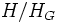# Normal core

VIEW RELATED: Analogues of this | Variations of this | Opposites of this |
View a complete list of semi-basic definitions on this wiki
This article defines a subgroup operator related to the subgroup property normal subgroup. By subgroup operator is meant an operator that takes as input a subgroup of a group and outputs a subgroup of the same group.

## Definition

QUICK PHRASES: largest normal subgroup inside, biggest normal subgroup inside, intersection of all conjugates

### Symbol-free definition

The normal core of a subgroup of a group is defined in the following equivalent ways:

### Definition with symbols

The normal core of a subgroup$H$ in a group$G$, denoted as$H_G$, is defined in the following equivalent ways:

• (Normal subgroup definition) As the subgroup generated by all normal subgroups$N$ of$G$ that lie inside$H$ i.e.:$H_G := \langle N : N \triangleleft G, N \le H \rangle$

• (Conjugate-intersection definition) As the intersection of all subgroups of the form$gHg^{-1}$ where$g$ varies over$G$, i.e.:$H_G := \bigcap_{g \in G} gHg^{-1}$

• (Group action definition) As the kernel of the action of$G$ on the coset space$G/H$ (see group acts on left coset space of subgroup by left multiplication):$H_G = \operatorname{ker} (G \to Sym(G/H))$

## Related notions

### Other operators involving approximating by normal subgroups

• Normal closure is the smallest normal subgroup of the whole group containing a given subgroup.
• Normalizer is the largest subgroup containing a given subgroup in which that subgroup is normal.

### Analogous operators in other structures

• Ideal core of a Lie subring: This is the analogous notion in Lie rings.
• Normal closure of a field extension: This is the precise corresponding notion under the correspondence given by the fundamental theorem of Galois theory. Note that normal core becomes normal closure because of the direction-reversing nature of the correspondence.
• Regular closure of a covering map: This is the precise corresponding notion under the correspondence between subgroups of the fundamental group and connected covers. Core becomes closure because of the direction-reversing nature of the correspondence.

## Related subgroup properties

### Image

The normal core is an operator that takes subgroups to subgroups. It is an idempotent operator and the fixed points are precisely the normal subgroups.

In other words, the normal core of any subgroup is a normal subgroup, and the normal core of a normal subgroup is itself.

### Inverse image of trivial subgroup

If the normal core of a subgroup is trivial, the subgroup is said to be a core-free subgroup.

## Quotient by normal core

Note that because normality satisfies the intermediate subgroup condition, the normal core of a subgroup is normal within the subgroup. That is, if$H$ is a subgroup of$G$,$H_G$ is a normal subgroup of$H$. Thus, we can talk of the quotient$H/H_G$. The relation between properties of$H$ and properties of$H/H_G$ are studied in the theory of the quotient-by-core.

## Computation

Further information: normal core-finding problem

The normal core of a subgroup in a group can be found computationally by invoking the membership testing problem as well as the group intersection problem. The part involving invocation of the membership testing problem can be thought of as a variant of the normality testing problem.